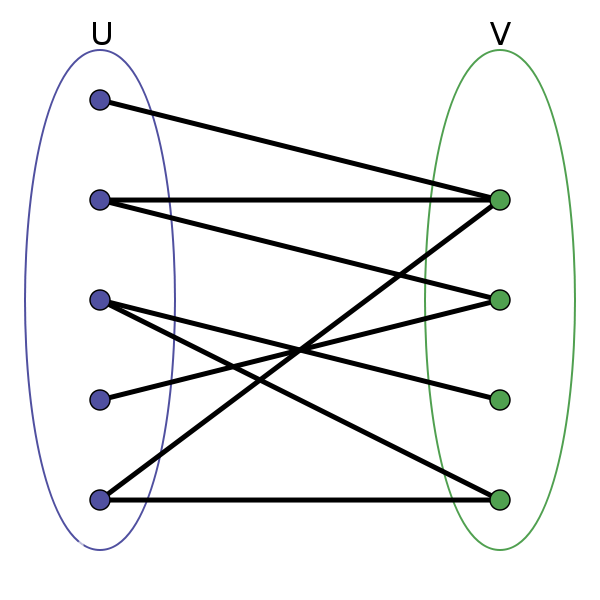2019/03/01 22:01

# 二分图

#### 区别二分图，关键是看点集是否能分成两个独立的点集。如下图所示# 匈牙利算法

//mk[x]表示点x所匹配的点，mark[x]表示x已被走过
bool sp(int x){
for(int i=0;i<edge[x].size();i++){
int v=eedge[x][i];
if(mark[v])continue;
mark[v]=1;
if(mk[v]==-1||sp(mk[v])){
mk[v]=x;
return true;
}
}
return false;
}
int solve(){
int ans=0;
memset(mk,-1,sizeof(mk));
for(int i=1;i<=n；i++){
memset(mark,0,sizeof(mark));
if(sp(i))ans++;
}
return ans;
}


## 二分图的最大团

0
0 收藏

### 作者的其它热门文章0 评论
0 收藏
0